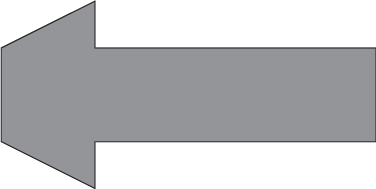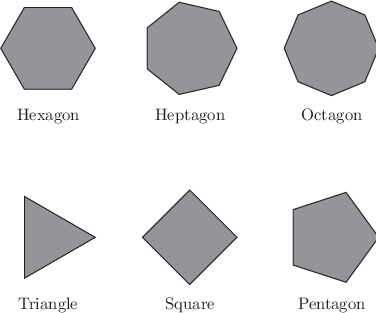# 10.7 The polygon command

Example: A simple polygon

In this simple example, we use Pyxplot’s polygon command to generate a geometric shape from a list of points.
points = [ [0,-1], [0, 1], [2, 2], [2,1], [8,1],[8,-1], [2,-1], [2,-2]]

polygon points with fillcol gray50 col blackExample: The first eight regular polygons

This example uses Pyxplot’s flow control commands, together with its list methods and the polygon command, to generate a diagram of the first eight regular polygons.
rotate(a) = matrix( [cos(a), -sin(a)],[sin(a),  cos(a)] )

subroutine makePolygon(Nsides, centre)
{
points = []
for i=0 to Nsides
{
call points.append(centre +rotate(i/Nsides*unit(rev)) * vector(1,0))
}
polygon points with fillcol gray50 col black
}

set nodisplay ; set multiplot

set texthalign center
set textvalign top

foreach datum x,y,Nsides,name in "–"
{
call makePolygon(Nsides,vector(x,y))
text name at x,y-1.25
}
0 0 3 Triangle
3 0 4 Square
6 0 5 Pentagon
0 4 6 Hexagon
3 4 7 Heptagon
6 4 8 Octagon
END

set display ; refresh